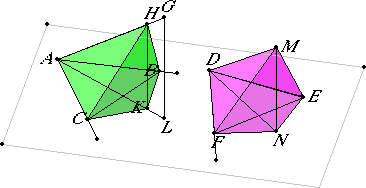# Proposition 35

If there are two equal plane angles, and on their vertices there are set up elevated straight lines containing equal angles with the original straight lines respectively, if on the elevated straight lines points are taken at random and perpendiculars are drawn from them to the planes in which the original angles are, and if from the points so arising in the planes straight lines are joined to the vertices of the original angles, then they contain with the elevated straight lines equal angles.

Let the angles BAC and EDF be two equal rectilinear angles, and from the points A and D let the elevated straight lines AG and DM be set up containing, with the original straight lines, equal angles respectively, namely, the angle MDE equal to the angle GAB and the angle MDF equal to the angle GAC.

XI.11

Take the points G and M at random on AG and DM. Draw GL and MN from the points G and M perpendicular to the plane through BA and AC and the plane through ED and DF, and let them meet the planes at L and N. Join LA and ND.

I say that the angle GAL equals the angle MDN.Make AH equal to DM, and draw HK through the point H parallel to GL.

XI.8

Since GL is perpendicular to the plane through BA and AC, therefore HK is also perpendicular to the plane through BA and AC.

I.12

Draw KC, NF, KB and NE from the points K and N perpendicular to the straight lines AC, DF, AB, and DE. Join HC, CB, MF, and FE.

I.47

Since the square on HA equals the sum of the squares on HK and KA, and the sum of the squares on KC and CA equals the square on KA, therefore the square on HA equals the sum of the squares on HK, KC, and CA.

But the square on HC equals the sum of the squares on HK and KC, therefore the square on HA equals the sum of the squares on HC and CA. Therefore the angle HCA is right. For the same reason the angle DFM is also right.

I.26

Therefore the angle ACH equals the angle DFM. But the angle HAC equals the angle MDF. Therefore MDF and HAC are two triangles which have two angles equal to two angles respectively, and one side equal to one side, namely, that opposite one of the equal angles, that is, HA equals MD, therefore they also have the remaining sides equal to the remaining sides respectively. Therefore AC equals DF.

I.4

Similarly we can prove that AB also equals DE. Since then AC equals DF, and AB equals DE, the two sides CA and AB equal the two sides FD and DE. But the angle CAB also equals the angle FDE, therefore the base BC equals the base EF, the triangle equals the triangle, and the remaining angles to the remaining angles. Therefore the angle ACB equals the angle DPE.

But the right angle ACK equals the right angle DFN, therefore the remaining angle BCK equals the remaining angle EFN. For the same reason the angle CBK also equals the angle FEN.

I.26

Therefore BCK and EFN are two triangles which have two angles equal to two angles respectively, and one side equal to one side, namely, that adjacent to the equal angles, that is, BC equals EF, therefore the remaining sides equal the remaining sides. Therefore CK equals FN.

I.4

But AC also equals DF, therefore the two sides AC and CK equal the two sides DF and FN, and they contain right angles. Therefore the base AK equals the base DN.

I.47

And, since AH equals DM, therefore the square on AH equals the square on DM. But the sum of the squares on AK and KH equals the square on AH, for the angle AKH is right, and the sum of the squares on DN and NM equals the square on DM, for the angle DNM is right, therefore the sum of the squares on AK and KH equals the sum of the squares on DN and NM. And of these the square on AK equals the square on DN, therefore the remaining square on KH equals the square on NM. Therefore HK equals MN.

I.8

And, since the two sides HA and AK equal the two sides MD and DN respectively, and the base HK equals the base MN, therefore the angle HAK equals the angle MDN.

Therefore, if there are two equal plane angles, and on their vertices there are set up elevated straight lines containing equal angles with the original straight lines respectively, if on the elevated straight lines points are taken at random and perpendiculars are drawn from them to the planes in which the original angles are, and if from the points so arising in the planes straight lines are joined to the vertices of the original angles, then they contain with the elevated straight lines equal angles.

Q.E.D.

# Corollary

From this it is clear that, if there are two equal plane angles, and if elevated straight lines set up on them which are equal and contain equal angles with the original straight lines respectively, then the perpendiculars drawn from their ends to the planes in which are the original angles equal one another.

## Guide

The situation described here occurs in the next proposition, and the corollary is used there to show two parallelepipeds have the same height.

Euclid’s proof is quite long. Various authors have substituted shorter ones.